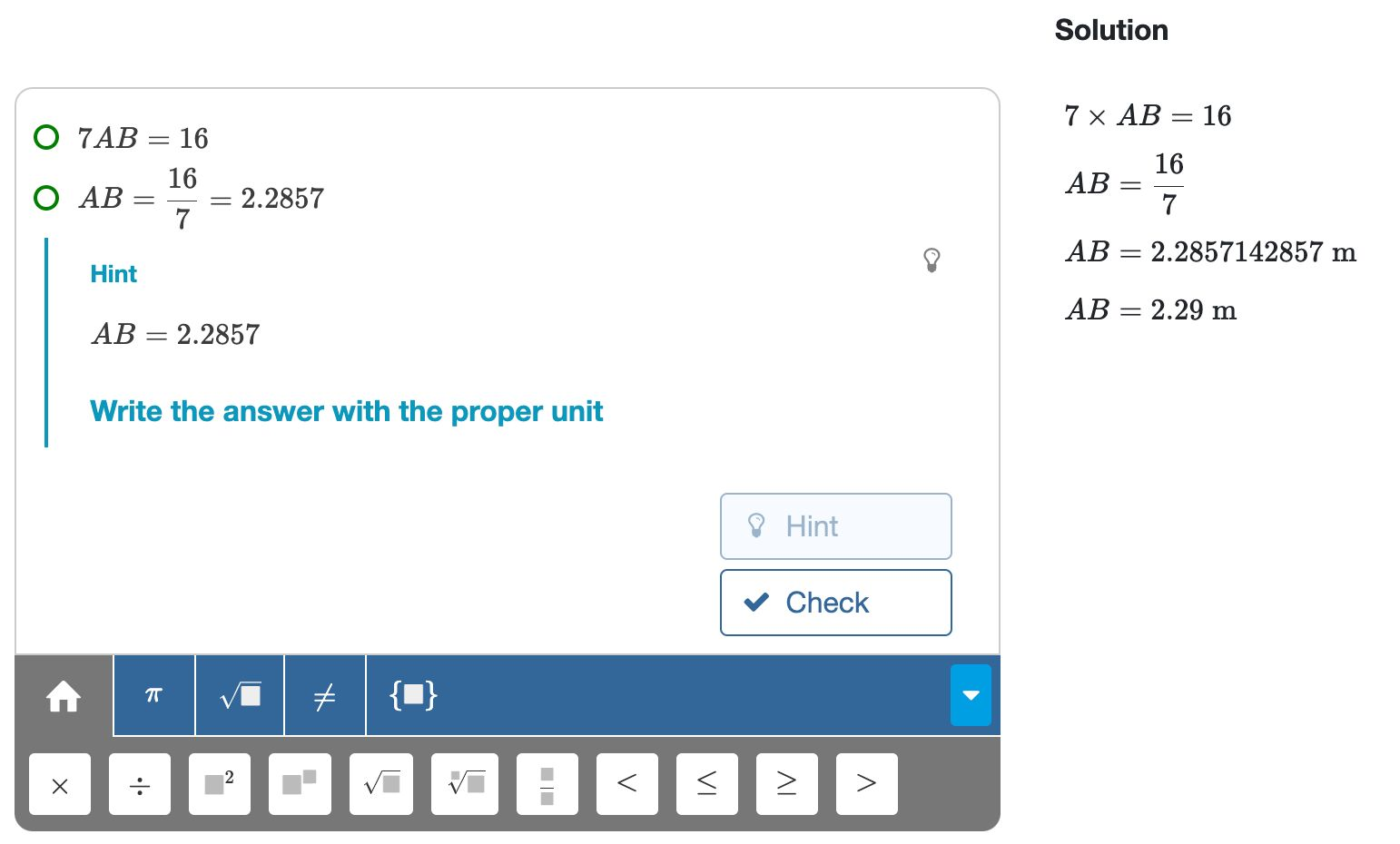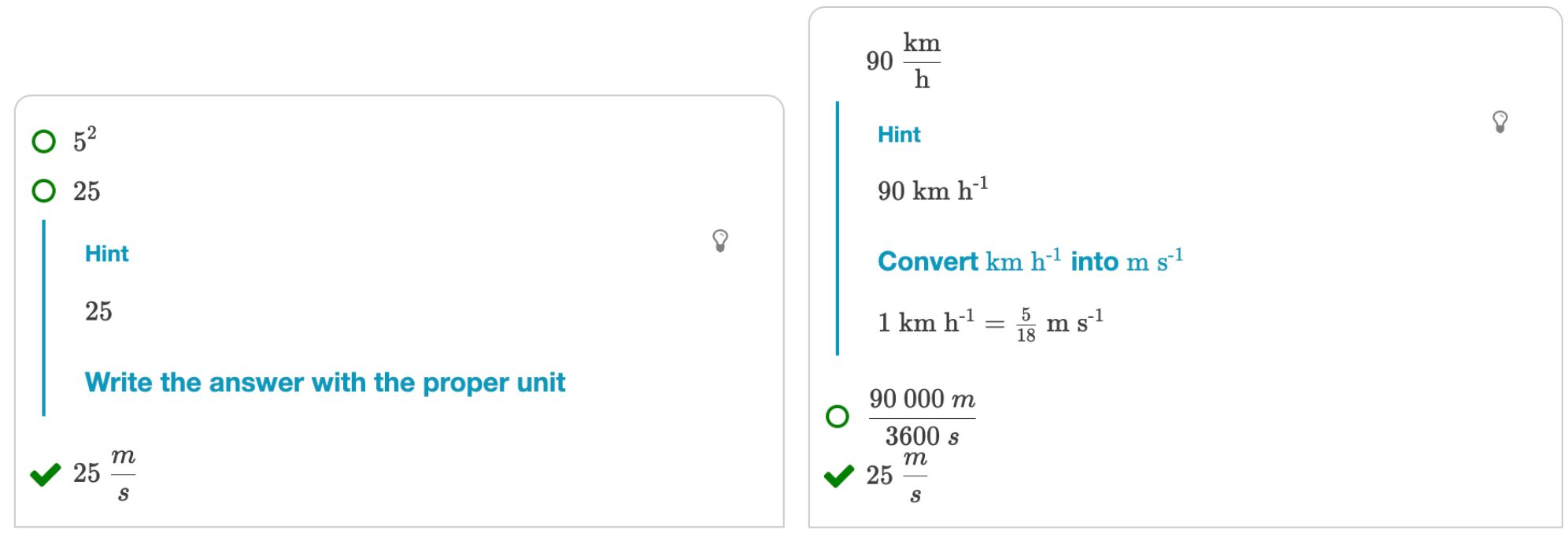We have seen how tasks define what problems students have to solve. However, students often have to do extra work after solving the task. Examples are:

• Numerically evaluating and possibly rounding the result
• Writing the result with the proper unit.
• Writing the result in scientific notation.

You can configure such post-processing through the Task Settings.Figure: Rounding and adding a unit after solving an equation. Left: an interactive session. Right: a worked solution.

You can open the Task Settings panel with the toggle above the Tasks bar. With the toggle enabled, extra options for accuracy and units appear below the taskbar. We will discuss the accuracy and the unit settings below.Figure: Task Settings toggle

## Accuracy settings

Click on the accuracy option to reveal its settings.Figure: Accuracy settings

The default setting is 'exact', meaning students should not approximate their answers. So students should keep roots and fractions if these cannot be written in an exact decimal form.

• The exact solution of $3x=10$ is $x=\frac{10}{3}$ or $x=3\frac{1}{3}$, but not $3.333333$.
• The exact solution of $2x=3$ is $x=\frac{3}{2}$, $x=1\frac{1}{2}$, or $x=1.5$.

Any other option than 'exact' implies that students must numerically approximate the result. We will discuss the various types of approximations below.

### Rounded to .. decimals

Students must round the answer to the prescribed number of decimal places.

Example: Round $\pi$ to two decimals

• $3.142\rightarrow$ intermediate step. Too many decimal places.
• $3.1\rightarrow$ incorrect. Not enough decimal places.
• $3.15\rightarrow$ incorrectly rounded.
• $3.14\rightarrow$ final answer

### Accurate to ... decimals

The answer must be accurate to at least the prescribed number of decimals.

Example: Write $\pi$ accurate to two decimals

• $3.142\rightarrow$ final answer
• $3.14\rightarrow$ final answer
• $3.1\rightarrow$ incorrect, not accurate enough.

### Significant figures

Significant figures are the number of digits in a value. For example, 3.1415 has five signification figures. This type of accuracy is expected in STEM problems where calculations usually depend on measured values.

Example: Approximate $\frac{5}{2}$ to three significant figures

• $2.5\rightarrow$ intermediate step. Not enough significant figures.
• $2.500\rightarrow$ intermediate step. Too many significant figures.
• $2.50\rightarrow$ final answer.

### Range

Sometimes you want to set the upper and lower bounds explicitly. An example is a length which students must measure with their ruler. Note that the range always includes the lower bound but excludes the upper bound.

## Units

You can use Multistep for calculations that involve quantities and units. Algebrakit recognizes the notations of many standard units and can convert one unit into another.

Just like rounding, adding the unit is something you usually do at the end of a derivation. This way of working, and some other settings, can be configured in the Unit Settings.Figure: Unit settings

The elements in the Unit Settings are divided into three categories, as indicated by the yellow labels in the image.

• (A) The unit of the result. If also the task has a unit, then it will be converted. Otherwise, it will be added. See the figure below.
• (B) Whether equivalent units are allowed as a final answer or if students need to convert these to the desired unit.
• (C) Is the unit optional or required? Is it allowed to postpone adding the unit toward the end of the derivation (which is the default behavior), or must it be written with every intermediate step?Figure: Left: Adding units at the end of a calculation. Right: converting units